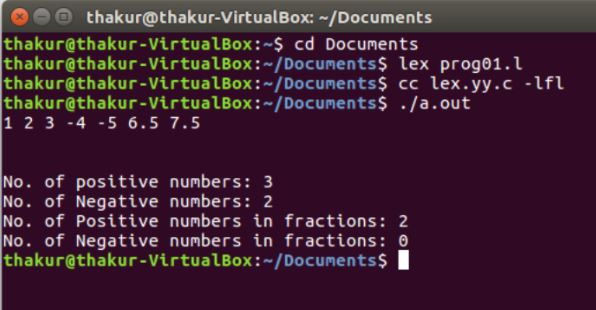# Lex program to Count the Positive numbers, Negative numbers and Fractions

Problem : Write a Lex program to count the positive numbers, negative numbers and fractions

Explanation :
FLEX (Fast Lexical Analyzer Generator) is a tool/computer program for generating lexical analyzers (scanners or lexers) written by Vern Paxson in C around 1987. Lex reads an input stream specifying the lexical analyzer and outputs source code implementing the lexer in the C programming language. The function yylex() is the main flex function which runs the Rule Section.

Prerequisite: Flex (Fast lexical Analyzer Generator)

Examples:

```Input: 2 -8 -2.5 8.5
Output: No. of positive numbers: 1
No. of Negative numbers: 1
No. of Positive numbers in fractions: 1
No. of Negative numbers in fractions: 1

Input: 1 2 3 -4 -5 6.5 7.5
Output: No. of positive numbers: 3
No. of Negative numbers: 2
No. of Positive numbers in fractions: 2
No. of Negative numbers in fractions: 0 ```

Implementation:

 `/* Lex program to Count the Positive numbers,  ` `      ``- Negative numbers and Fractions  */` ` `  `%{ ` `     ``/* Definition section */` `    ``int` `postiveno=0; ` `    ``int` `negtiveno=0; ` `    ``int` `positivefractions=0; ` `    ``int` `negativefractions=0; ` `%} ` ` `  `/* Rule Section */` `DIGIT [0-9] ` `%% ` ` `  `\+?{DIGIT}+             postiveno++; ` `-{DIGIT}+               negtiveno++; ` ` `  `\+?{DIGIT}*\.{DIGIT}+   positivefractions++; ` `-{DIGIT}*\.{DIGIT}+     negativefractions++; ` `. ;    ` `%% ` ` `  `// driver code ` `int` `main() ` `{ ` `    ``yylex(); ` `    ``printf``(``"\nNo. of positive numbers: %d"``, postiveno); ` `    ``printf``(``"\nNo. of Negative numbers: %d"``, negtiveno); ` `    ``printf``(``"\nNo. of Positive numbers in fractions: %d"``, positivefractions); ` `    ``printf``(``"\nNo. of Negative numbers in fractions: %d\n"``, negativefractions); ` `    ``return` `0; ` `} `

Output:Whether you're preparing for your first job interview or aiming to upskill in this ever-evolving tech landscape, GeeksforGeeks Courses are your key to success. We provide top-quality content at affordable prices, all geared towards accelerating your growth in a time-bound manner. Join the millions we've already empowered, and we're here to do the same for you. Don't miss out - check it out now!

Previous
Next